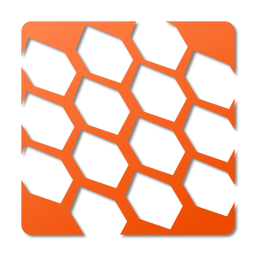#NetFabric.Numerics 1.0.0-beta04Prefix Reserved
.NET 7.0
This is a prerelease version of NetFabric.Numerics.
There is a newer prerelease version of this package available.
See the version list below for details.
`dotnet add package NetFabric.Numerics --version 1.0.0-beta04`
`NuGet\Install-Package NetFabric.Numerics -Version 1.0.0-beta04`
This command is intended to be used within the Package Manager Console in Visual Studio, as it uses the NuGet module's version of Install-Package.
`<PackageReference Include="NetFabric.Numerics" Version="1.0.0-beta04" />`
For projects that support PackageReference, copy this XML node into the project file to reference the package.
`paket add NetFabric.Numerics --version 1.0.0-beta04`
`#r "nuget: NetFabric.Numerics, 1.0.0-beta04"`
#r directive can be used in F# Interactive and Polyglot Notebooks. Copy this into the interactive tool or source code of the script to reference the package.
```// Install NetFabric.Numerics as a Cake Addin

// Install NetFabric.Numerics as a Cake Tool
#tool nuget:?package=NetFabric.Numerics&version=1.0.0-beta04&prerelease```

## NetFabric.Numerics

NetFabric.Numerics provides strongly-typed implementations for cartesian and polar coordinates, and operations for 2D and 3D vectors and quaternions.

WARNING: NetFabric.Numerics makes use of generic math features only available in .NET 7 and C# 11. Make sure you are using a compatible version of the framework before using this library.

``````using NetFabric.Numerics;

// Create points
var point2DInteger = new Cartesian2.Point<int>(10, 20);
var point2DFloat = new Cartesian2.Point<float>(10.0f, 20.0f);
var point2DDouble = new Cartesian2.Point<double>(10.0, 20.0);

var pointPolarDegreesFloat = new Polar.Point<Degrees, float, float>(
Angle<Degrees, float>.Right, // 90 degrees azimuth
new Angle<Radians, double>(double.Pi), // 180 degrees azimuth

var point3DInteger = new Cartesian3.Point<int>(10, 20, 30);
var point3DFloat = new Cartesian3.Point<float>(10.0f, 20.0f, 30.0f);
var point3DDouble = new Cartesian3.Point<double>(10.0, 20.0, 30.0);

var pointSphericalDegreesFloat = new Spherical.Point<Degrees, float, float>(
Angle<Degrees, float>.Zero, // 0 degrees azimuth
Angle<Degrees, float>.Right, // 90 degrees zenith
10.0f); // 90 degrees

// Create quaternions
var quaternionFloat = new Cartesian3.Quaternion<float>(1.0f, 2.0f, 3.0f, 4.0f);
var quaternionDouble = Cartesian3.Quaternion.FromYawPitchRoll<double>(
Angle<Radians, double>.Zero, // 0 degrees yaw
Angle<Radians, double>.Zero, // 0 degrees pitch
Angle<Radians, double>.Right); // 90 degrees roll

// Perform math operations
var vector3DDouble = point3DDouble - new Cartesian3.Point<double>(1.0, 1.0, 1.0);
var point3DTransformed = point3DDouble + vector3DDouble;

// Conversions
var convertToFloatChecked = Cartesian3.Point<float>.CreateChecked(point3DDouble); // throws if value is out of range
var convertToFloatSaturated = Cartesian3.Point<float>.CreateSaturating(point3DDouble); // saturate if value is out of range
var convertToFloatTruncated = Cartesian3.Point<float>.CreateTruncating(point3DDouble); // truncate if value is out of range
``````

### Credits

The following open-source projects are used to build and test this project:

Product Compatible and additional computed target framework versions.
.NET net7.0 is compatible.  net7.0-android was computed.  net7.0-ios was computed.  net7.0-maccatalyst was computed.  net7.0-macos was computed.  net7.0-tvos was computed.  net7.0-windows was computed.  net8.0 was computed.  net8.0-android was computed.  net8.0-ios was computed.  net8.0-maccatalyst was computed.  net8.0-macos was computed.  net8.0-tvos was computed.  net8.0-windows was computed.
Compatible target framework(s)

### NuGet packages (2)

Showing the top 2 NuGet packages that depend on NetFabric.Numerics:

NetFabric.Numerics.GeographyPackage Description

NetFabric.Numerics.GeodesyPackage Description

### GitHub repositories

This package is not used by any popular GitHub repositories.